>

## Chapter 7 Statistics R.D. Sharma Solutions for Class 10th Math Exercise 7.3

1. The following table gives the distribution of total household expenditure (in rupees) of manual workers in a city.Find the average expenditure (in rupees) per household.

Solution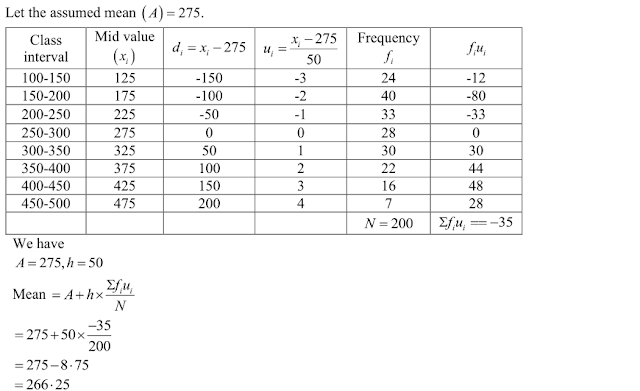2. A survey was conducted by a group of students as a part of their environment awareness program, in which they collected the following data regarding the number of plants in 20 houses in a locality. Find the mean number of plants per house .
Number of plants: 0-2 2-4 4-6 6-8 8-10 10-12 12-14
Number of houses: 1  2  1 5 6  2  3
Which method did you use for finding the mean, and why?

Solution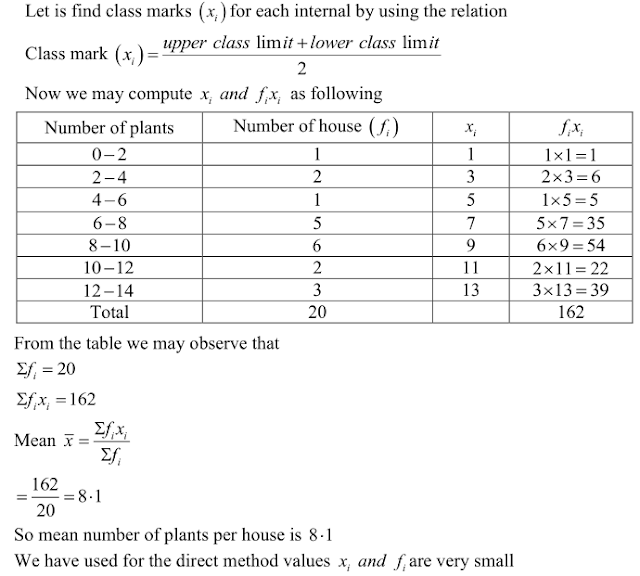3. Consider the following distribution of daily wages of 50 workers of a factory
Daily wages (in Rs). 100-120 120-140 140-160 160-180 180-200
Number of workers: 12 14  8  6  10
Find the mean daily wages of the workers of the factory by using an appropriate method.

Solution4. Thirty women were examined in a hospital by a doctor and the number of heart beats per
minute recorded and summarized as follows. Find the mean heart beats per minute for these
women, choosing a suitable method.
Number of heat
beats per minute: 65 - 68 68 - 71 71 - 74 74 - 77 77 - 80 80 - 83 83 -86
Number of women: 2  4   3  8  7   4  2

SolutionFind the mean of each of the following frequency distributions: (5 − 14)
5. Class interval: 0-6 6-12 12-18 18-24 24-30
Frequency:  6  8  10  9  7

Solution6. Class interval: 50 - 70 70 - 90 90 - 110 110 - 130 130 - 150 150 – 170
Frequency: 18 12 13 27 8 22

Solution7. Class interval: 0-8 8- 16 16- 24 24-32 32-40
Frequency: 6 7 10 8 9

Solution8. Class interval: 0-6 6-12 12-18 18-24 24-30
Frequency: 7 5 10 12 6

Solution9. Class interval: 0- 10 10- 20 20-30 30-40 40-50
Frequency: 9 12 15 10 14

Solution10. Class interval: 0-8 8- 16 16-24 24-32 32-40
Frequency: 5 9 10 8 8

Solution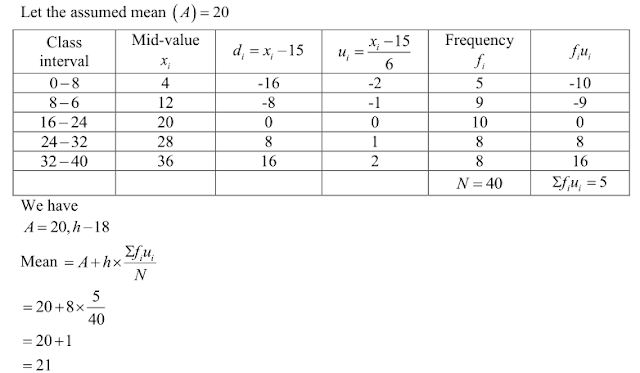11. Class interval: 0-8 8- 16 16- 24 24-32 32-40
Frequency: 5 6 4 3 2

Solution12. Class interval: 10-30 30-50 50-70 70-90 90-110 110- 130
Frequency: 5  8 12 20 3 2

Solution13. Class interval: 25-35 35-45 45-55 55 - 65 65 -75
Frequency: 6 10 8 12 4

Solution14. Classes: 25 -29 30-34 35-39 40-44 45-49 50-54 55-59
Frequency: 14 22 16 6 5 3 4

Solution15. For the following distribution, calculate mean using all suitable methods:
Size of item: 1-4 4-9 9- 16 16-27
Frequency: 6 12 26 20

Solution16. The weekly observations on cost of living index in a certain city for the year 2004 - 2005 are given below. Compute the weekly cost of living index.Solution17. The following table shows the marks scored by 140 students in an examination of a certain paper:
Marks: 0-10 10-20 20-30 30-40 40-50
Number of students: 20 24 40 36 20
Calculate the average marks by using all the three methods: direct method, assumed mean deviation and shortcut method.

Solution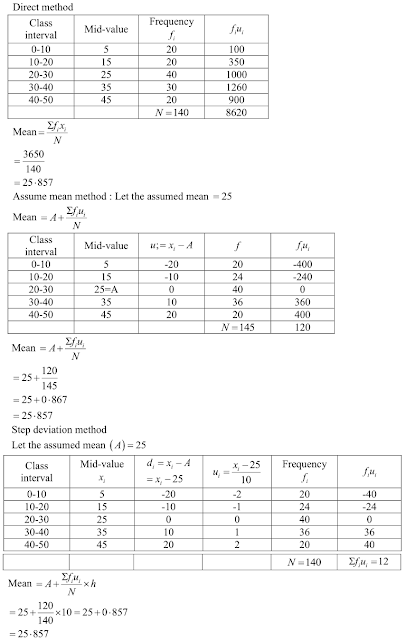18. The mean of the following frequency distribution is 62.8 and the sum of all the frequencies is 50. Compute the missing frequency f1 and f2 .
Class: 0-20 20-40 40-60 60-80 80-100 100–120
Frequency: 5 f1 10 f2 7 8

Solution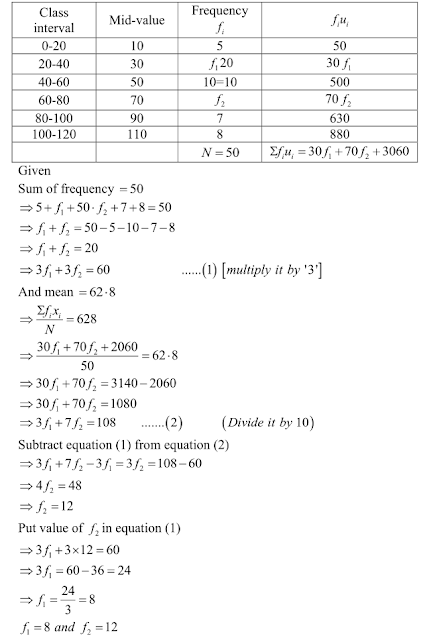19. The following distribution shows the daily pocket allowance given to the children of a multistorey building. The average pocket allowance is Rs 18.00. Find out the missing frequency.
Class interval: 11-13 13-15 15-17 17-19 19-21 21-23 23-25
Frequency: 7 6 9 13 - 5 4

Solution20. If the mean of the following distribution is 27, find the value of p.
Class: 0-10 10-20 20-30 30-40 40-50
Frequency: 8  p 12 13 10

Solution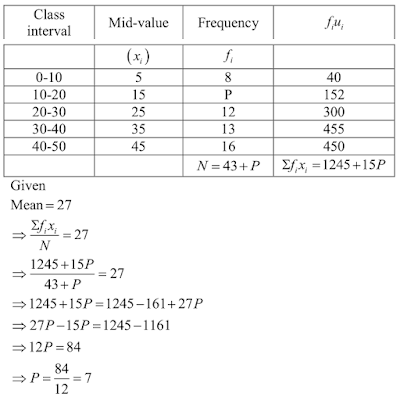21. In a retail market, fruit vendors were selling mangoes kept in packing boxes. These boxes
contained varying number of mangoes. The following was the distribution of mangoes
according to the number of boxes.
Number of mangoes: 50-52 53-55 56-58 59-61 62-64
Number of boxes: 15 110 135 115 25
Find the mean number of mangoes kept in a packing box. Which method of finding the
mean did you choose ?

Solution22. The table below shows the daily expenditure on food of 25 households in a locality
Daily expenditure (in Rs): 100-150 150-200 200-250 250-300 300-350
Number of households: 4 5 1 2  2 2
Find the mean daily expenditure on food by a suitable method.

Solution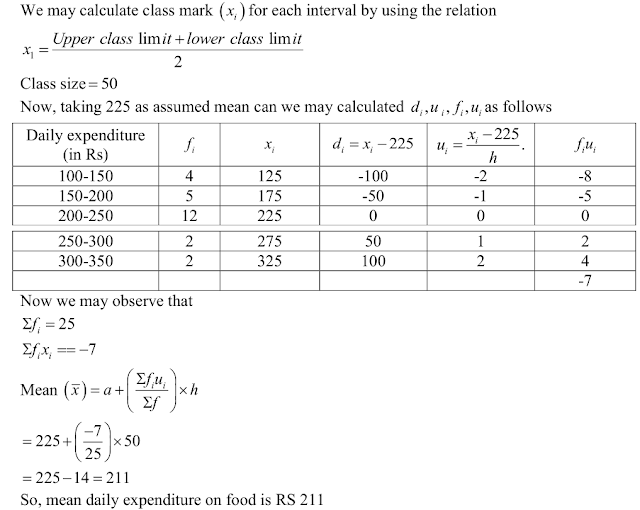23. To find out the concentration of SO2 in the air (in parts per million, i.e., ppm), the data was
collected for 30 localities in a certain city and is presented below .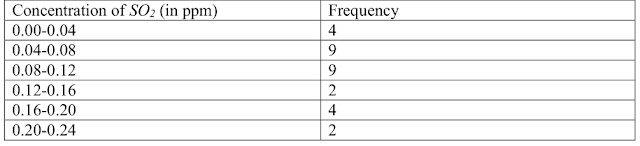Find the mean concentration of SOin the air.

Solution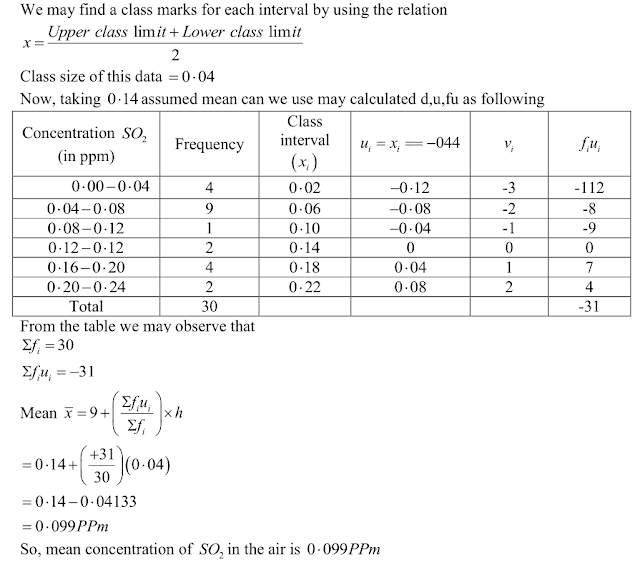24. A class teacher has the following absentee record of 40 students of a class for the whole term. Find the mean number of days a student was absent.
Number of days:  0-6 6-10 10-14 14-20 20-28 28-38 38-40
Number of students: 11 10  7  4  4  3 1

Solution25. The following table gives the literacy rate (in percentage) of 35 cities. Find the mean literacy rate.
Literacy rate (in %): 45-55 55-65 65-75 75-85 85-95
Number of cities: 3 10 11  8 3

Solution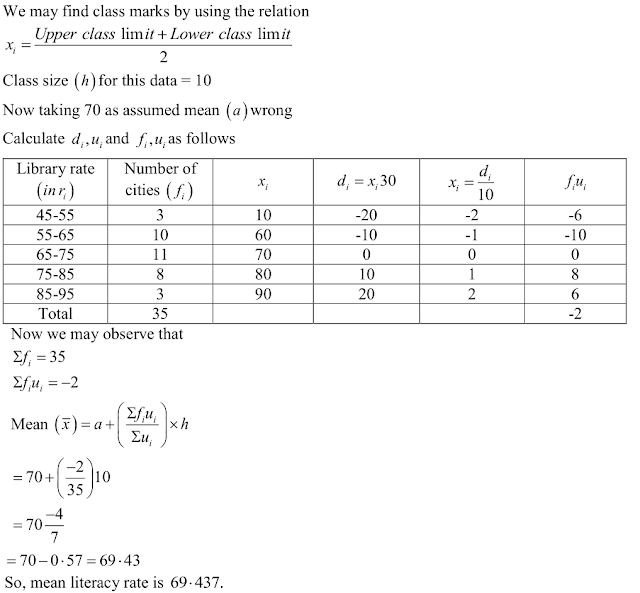26 . The following is the cummlative frequency distribution (of less than type) of 100 persons each of age 20 years and above . Determine the mean age .
Age below (in years) : 30  40    50   60  70    80
Number of persons :  100 220 350 760  950 1000

Solution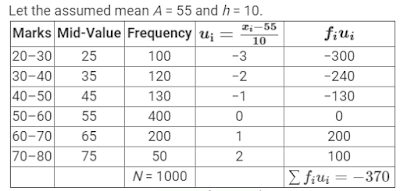We know that mean , x̅ = A + h(1/N Σfiui)
Now, we have N = Σfi = 1000, h = 10, A = 55, Σfiui = - 370
x̅ = 55 + 10 [1/100 × (-370)]
= 55 - 3.7
= 51.3 years

27. If the mean of the following frequency distribution is 18, find the missing frequency .
Class intervals : 11-13 13 -15 15 -17 17-19 19-21 21-23 23-25
Frequency : 3 6  9 13  f  5 4

Solution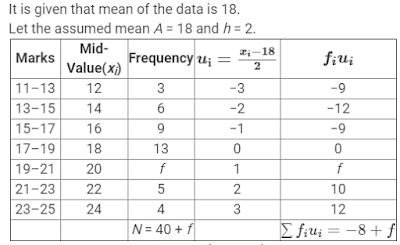We know that mean , x̅ = A + h(1/N Σfiui)
Now, we have N = Σfi = 40 + f, h = 2, A = 18, Σfiui = -8 + f
= 18 = 18 + 2 [1/(40+f) × (-8 + f)
⇒ 0 = -16+2f/40+f
⇒ -16 + 2f = 0
⇒ f = 8

28. Find the missing frequencies in the following distribution, if the sum of the frequencies is 120 and the mean is 50.
Class: 0-20 20-40 40-60 60-80 80-100
Frequency :  17 fi  32 f2 19

Solution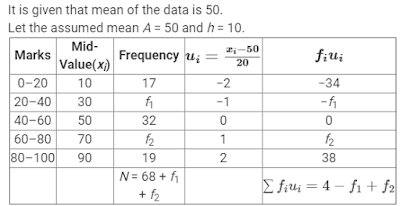We know that mean , x̅ = A + h(1/N Σfiui)
Now, we have N = 68 + f1 + f2, h = 20, A = 50, Σfiui = 4 - f1 + f2.
50 = 50 + 20 (4-f1+f2/68+f1+f2)
⇒ f1 - f2 = 4
⇒ f1 = 4 + f2 .....(1)
Now, N = 68 + f1 + f2 = 120
f1 + f2 = 120 - 68 = 52
⇒ 4 + f2 + f2 = 52 [Using (1)]
⇒ f2 = 24
so,
f1 = 4 + 24 = 28

29. The daily incomes of a sample of 50 employees are tabulated as follows :
Income: 1-200 201-400 401-600 601-800
No.of employees : 14  15  14  7
Find the mean daily income of employees .

Solution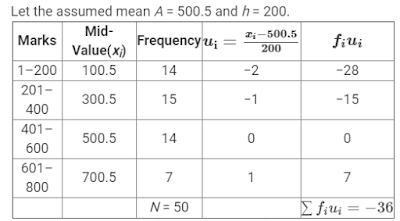We know that mean , x̅ = A + h(1/N Σfiui)
Now, we have N = Σfi = 50, h = 200, A = 500.5, Σfiui = -36
x̅ = 500.5 + 200 [1/50 × (-36)]
= 500.5 - 144
= 356.5
X